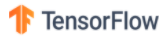# Catch up on the latest AI articles# Can You Learn How To Teach?

3 main points
✔️ Learning to Teach accelerates learning
✔️ Meta-learning generates synthetic data that allows the network to learn faster

✔️ Learning of neural networks 9 times faster

Donald J. Hejna IIIPieter AbbeelLerrel Pinto
(Submitted on 3 Mar 2020 (v1), last revised 31 Aug 2020 (this version, v2))

Subjects: Machine Learning (cs.LG); Artificial Intelligence (cs.AI); Robotics (cs.RO); Machine Learning (stat.ML)## Introduction

Can you learn to teach?

The paper presented in this article is a study that addresses this challenging question. This question is similar but different from general meta-learning. Much of the existing meta-learning is focused on "learning how to learn," as it is described as "learning to learn.

In other words, the biggest problem is "how well can we learn from the data we are given".

If I had to compare it to studying.
When a textbook is given to students, how much more knowledge can they gain from it?
When students are given a task, how well can they solve it?
That was the goal.

In other words, the emphasis was on refining the "learners". However, is it really only the learners who matter? As long as a student excels, does it matter if the textbook or assignment given to that student isn't excellent? Intuitively, I wouldn't say that's the case. Of course, a good student can gain knowledge successfully from a textbook, no matter how difficult it is to understand. However, students will still learn more efficiently if they are given a textbook that is easy to understand.

Generative Teaching Networks (GTNs), introduced in this article, are a true "learning how to teach" method that allows us to create "easy-to-understand textbooks" in this analogy.